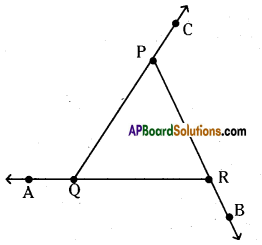AP State Syllabus AP Board 6th Class Maths Solutions Chapter 8 Basic Geometric Concepts Unit Exercise Textbook Questions and Answers.

## AP State Syllabus 6th Class Maths Solutions 8th Lesson Basic Geometric Concepts Unit ExerciseQuestion 1.
In the given figure, measure the length of AC. Check whether
i) AB + AC > AC
ii) AC > AD – DC
Solution:
In the given figure, AB = 4.2 cm; BC = 5.5 cm
AC = 5.4 cm; CD = 3 cm; AD = 4 cm.
i) AB + AC = 4.2 + 5.4 = 9.6 cm > 5.4 cm
AB + AC > AC
ii) AD – DC = 4 – 3 = 1 cm < 5.4 cm

Question 2.
Draw a line segment $$\overline{\mathbf{A B}}$$. Put a point C in between $$\overline{\mathbf{A B}}$$. Extend $$\overline{\mathbf{C B}}$$ upio D such that CD > AB. Now check whether AC and BD are equal length.
Solution: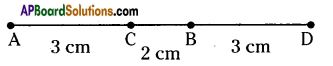Let draw a line $$\overline{\mathbf{A B}}$$ = 5 cm and mark a point C on $$\overline{\mathbf{A B}}$$ such that $$\overline{\mathbf{A C}}$$ = 3 cm (or) $$\overline{\mathbf{BC}}$$ = 2 cm Extend $$\overline{\mathbf{C B}}$$ up to D such that $$\overline{\mathbf{C D}}$$ = 5 cm ($$\overline{\mathbf{C B}}$$ = 2 cm, $$\overline{\mathbf{B D}}$$ = 3 cm)
∴ $$\overline{\mathbf{A C}}$$ = 3cm, $$\overline{\mathbf{C B}}$$ = 2cm, $$\overline{\mathbf{B D}}$$ = 3cm
∴ $$\overline{\mathrm{AC}}=\overline{\mathrm{BD}}$$ = 3cm.Question 3.
Draw an angle ∠AOB as m∠AOB = 40°. Draw an angle ∠BOC such that ∠AOC = 90°
Check whether m∠AOB + m∠BOC = m∠AOC.
Sol. Draw an angle ∠AOB = 40° and ∠AOC = 90° on the same ray $$\overrightarrow{\mathrm{OA}}$$
Now, measure ∠AOB = 40° and ∠BOC = 50°
∴ m∠AOB + m∠BOC = 40° + 50 = 90°
m∠AOB + m∠BOC = 90° = m∠AOC
∴ m∠AOB + m∠BOC = m∠AOC.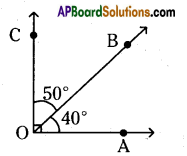Question 4.
Draw an angle ∠XYZ as m∠XYZ = 62°. Measure the exterior angle ∠XYZ.
Solution: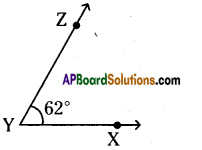Draw angle ∠XYZ = 62° (Interior)
Now, measure the exterior angle ∠XYZ.
∴ Exterior angle of ∠XYZ = 298°Question 5.
Match the following.
1. Set square — A) to measure angles
2. Protractor — B) to measure the lengths of line segments
3. Divider — C) to draw parallel lines
Sol. 1) Set square — C) to draw parallel lines
2) Protractor — A) to measure angles
3) Divider — B) to measure the lengths of line segments

Question 6.
List out the letters of English alphabet (capital letters) which consist of right angles.
Solution:
The letters which consists of right angles in English alphabet are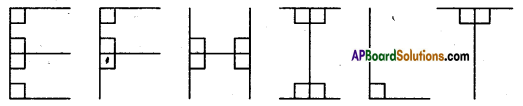Question 7.
Measure the angles ∠AQP, ∠CPR, ∠BRQ.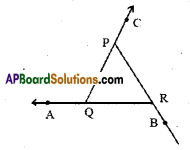Find m∠AQP, m∠CPR, m∠BRQ.
Solution:
In the given figure, measure the angles ∠AQR, ∠CPR, ∠BRQ
∠AQP = 120°
∠CPR = 120°
and ∠BRQ = 120°
∴ m∠AQP = 120°; m∠CPR = 120°; m∠BRQ = 120°